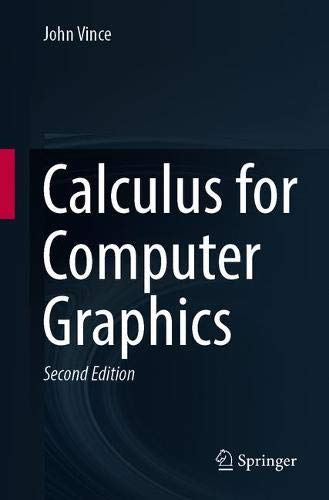• Title: Calculus for Computer Graphics, 2nd Edition
• Author: John Vince
• Length: 303 pages
• Edition: 2nd ed. 2019
• Language: English
• Publisher: Springer
• Publication Date: 2019-04-06
• ISBN-10: 3030113752
• ISBN-13: 9783030113759

## Description

Students studying different branches of computer graphics have to be familiar with geometry, matrices, vectors, rotation transforms, quaternions, curves and surfaces and as computer graphics software becomes increasingly sophisticated, calculus is also being used to resolve its associated problems.

In this 2nd edition, the author extends the scope of the original book to include applications of calculus in the areas of arc-length parameterisation of curves, geometric continuity, tangent and normal vectors, and curvature. The author draws upon his experience in teaching mathematics to undergraduates to make calculus appear no more challenging than any other branch of mathematics. He introduces the subject by examining how functions depend upon their independent variables, and then derives the appropriate mathematical underpinning and definitions. This gives rise to a function’s derivative and its antiderivative, or integral. Using the idea of limits, the reader is introduced to derivatives and integrals of many common functions. Other chapters address higher-order derivatives, partial derivatives, Jacobians, vector-based functions, single, double and triple integrals, with numerous worked examples, and over a hundred and seventy colour illustrations.

This book complements the author’s other books on mathematics for computer graphics, and assumes that the reader is familiar with everyday algebra, trigonometry, vectors and determinants. After studying this book, the reader should understand calculus and its application within the world of computer graphics, games and animation.